• 利此用代码进行图像的连通域的提取，简单通俗易懂，很好的选择
• 不仅判断图的连通性，而且程序还能求出该图的连通块数，并指出每个顶点分别属于哪个连通块。
• 车牌的matlab连通区域的提取，以前做的，连通区域提取
• MATLAB二维/三维二值图像连通域的快速标记算法。避开MATLAB函数库，方便改写为C/C++。
• 使用联通区域根据灰度图和二值图查找物体，用框框出来，并画出质心位置，并计算出符合一定规则的点，程序注释清楚
• matlab实现了二值图中连通区域数的统计，（不是使用的belabel函数完全自己书写代码），并将统计数在原图上显示
• Matlab中有bwlabel\bwboundaries两个连通分量标记函数 bwlabel（二维0-1） 作用：Label connected components in 2-D binary image 语法 L = bwlabel(BW) L = bwlabel(BW,conn) [L,n] = bwlabel(___) 说明 L...
Matlab中有bwlabel\bwboundaries两个连通分量标记函数
bwlabel（二维0-1）
作用：Label connected components in 2-D binary image
语法 L = bwlabel(BW) L = bwlabel(BW,conn) [L,n] = bwlabel(___)
说明
L = bwlabel(BW)返回标签矩阵L，其中包含BW中找到的8个连接对象的标签。 L = bwlabel(BW,conn)返回一个标签矩阵，其中conn指定连接，4或者8。 [L,n] = bwlabel()也返回n，即BW中找到的连接对象的数量。
对于bwlabel来说，其作用大致如下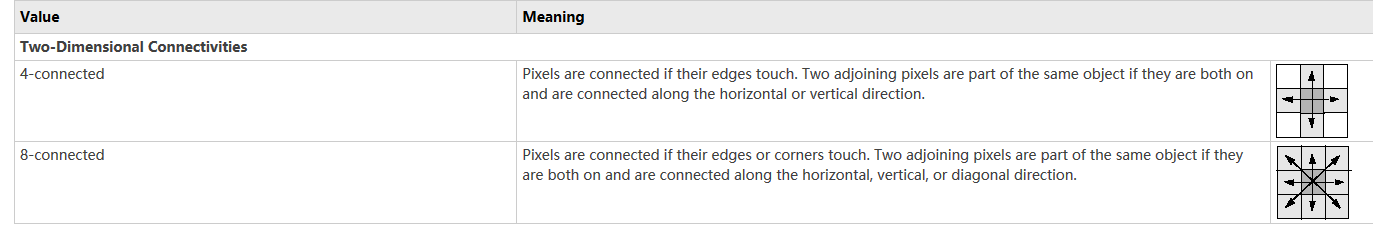bwlabeln（多维）
作用 Label connected components in binary image
语法 L = bwlabeln(BW) L = bwlabeln(BW,conn) [L,n] = bwlabeln(___)
说明
L = bwlabeln(BW) returns a label matrix, L, containing labels for the connected components in BW.
L = bwlabeln(BW,conn) returns a label matrix, where conn specifies the connectivity.
[L,n] = bwlabeln(___) also returns n, the number of connected objects found in BW.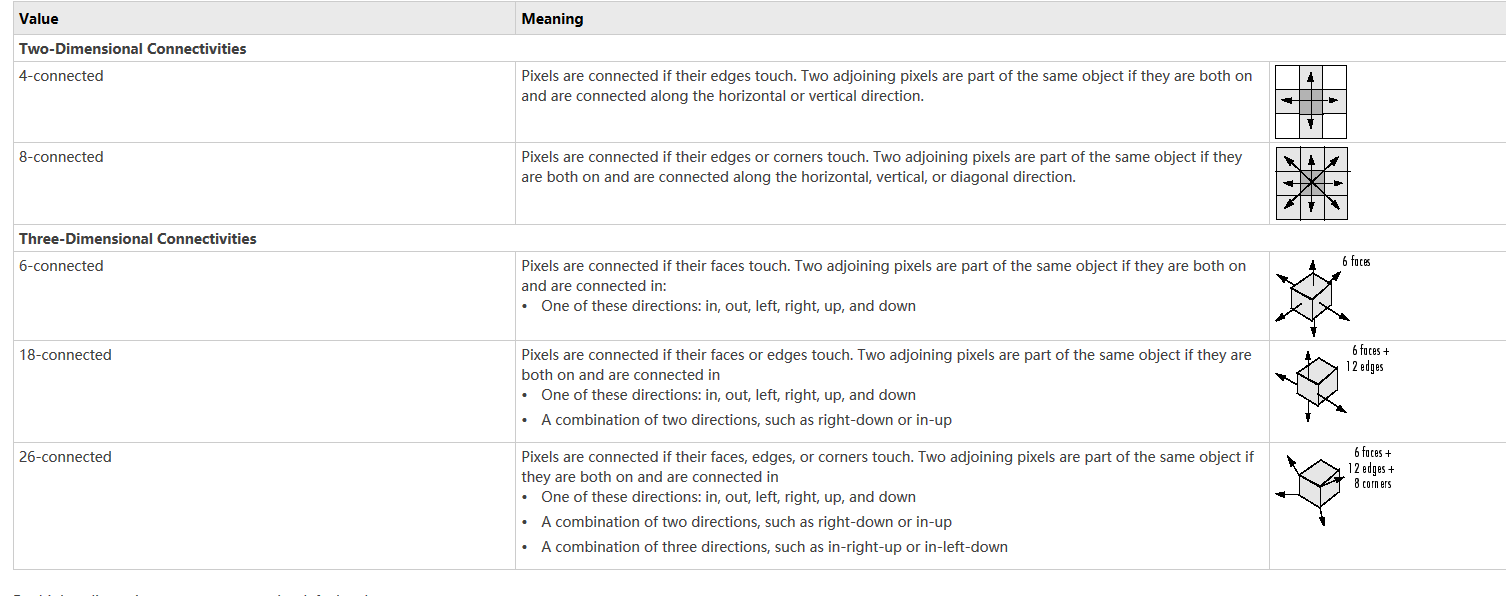bwboundaries
意译为：跟踪二进制图像中的区域边界
语法 B = bwboundaries(BW) B = bwboundaries(BW,conn) B = bwboundaries(BW,conn,options) [B,L]= bwboundaries(___) [B,L,n,A] = bwboundaries(___)
BW — Input binary image conn — Pixel connectivity ,8 (default) | 4 options — Determine whether to search for both parent and child boundaries,‘holes’ (default) | ‘noholes’ B — Row and column coordinates of boundary pixels(边界像素的行坐标和列坐标) L — Label matrix n — Number of objects found A — Parent-child dependencies between boundaries and holes(square, sparse, logical matrix.正方形，稀疏，逻辑矩阵) 说明 B = bwboundary (BW)在二值图像BW中，追踪物体的外部边界，以及物体内部的孔边界。bwboundary也向下延伸到最外层的对象(父对象)并跟踪其子对象(父对象完全包围的对象)。返回边界像素位置的单元格数组B。 B = bwboundary (BW,conn)跟踪对象的外部边界，其中conn指定在跟踪父和子边界时使用的连接。 B = bwboundary (BW,conn,options)跟踪对象的外部边界，其中的选项要么是“hole”，要么是“noholes”，指定是否希望包含其他对象内部的hole边界。 [B,L]= bwboundaries()返回一个标签矩阵L，其中标记了对象和孔。 [B,L,n,A] = bwboundaries()(_)返回找到的对象数量n和邻接矩阵A。
例子
//在图像上覆盖区域边界

//Convert grayscale image to binary image using local adaptive thresholding.
BW = imbinarize(I);

//Calculate boundaries of regions in image and overlay the boundaries on the image.
[B,L] = bwboundaries(BW,'noholes');

imshow(label2rgb(L, @jet, [.5 .5 .5]))
hold on
for k = 1:length(B)
boundary = B{k};
plot(boundary(:,2), boundary(:,1), 'w', 'LineWidth', 2)
end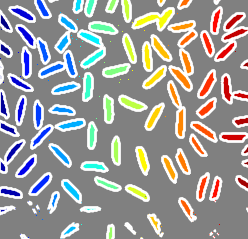//在图像上覆盖区域边界，并用区域号标注

//Calculate boundaries of regions in the image.

[B,L,N,A] = bwboundaries(BW);

//Display the image with the boundaries overlaid.
//Add the region number next to every boundary (based on the label matrix). Use the zoom tool to read individual labels.

imshow(BW); hold on;
colors=['b' 'g' 'r' 'c' 'm' 'y'];
for k=1:length(B),
boundary = B{k};
cidx = mod(k,length(colors))+1;
plot(boundary(:,2), boundary(:,1),...
colors(cidx),'LineWidth',2);

%randomize text position for better visibility
rndRow = ceil(length(boundary)/(mod(rand*k,7)+1));
col = boundary(rndRow,2); row = boundary(rndRow,1);
h = text(col+1, row-1, num2str(L(row,col)));
set(h,'Color',colors(cidx),'FontSize',14,'FontWeight','bold');
end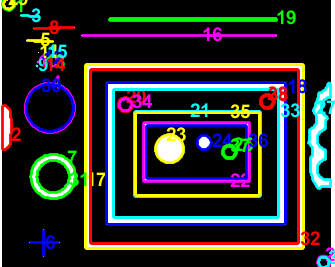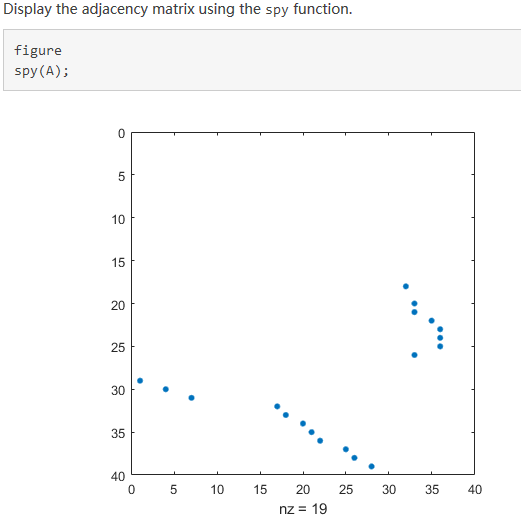//以红色显示对象边界，以绿色显示孔边界

//Calculate boundaries.

[B,L,N] = bwboundaries(BW);
//Display object boundaries in red and hole boundaries in green.

imshow(BW); hold on;
for k=1:length(B),
boundary = B{k};
if(k > N)
plot(boundary(:,2), boundary(:,1), 'g','LineWidth',2);
else
plot(boundary(:,2), boundary(:,1), 'r','LineWidth',2);
end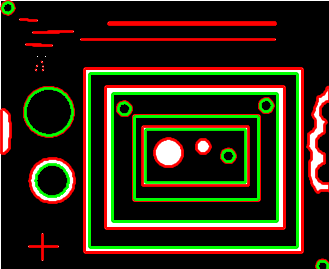好用的网站：https://ww2.mathworks.cn/help/images/ref/bwboundaries.html?s_tid=doc_ta 比买书有用多了，我买过一本matlab完全自学一本通，其实还不如这个网站来得直接。 openCV上也有这样得功能函数。
展开全文• matlab提取最大联通区域的程序，用的是matlab自带的图，可以直接在matlab上运行，用的matlab2018。有问题可以留言
• matlab，可以生成任意大小的连通矩阵，设置连通程度，可用于生成通信节点拓扑图
• matlab 连通区域 显示
I = imread('1.1.jpg');%ROI 分割区域
BW = im2bw(I, graythresh(I));%二值化
[B,L] = bwboundaries(BW,'noholes');%寻找边缘，不包括孔
imshow('1.jpg');%原图
hold on
for k = 1:length(B)
boundary = B{k};

plot(boundary(:,2), boundary(:,1), 'w', 'LineWidth', 2)
end%整个循环表示的是描边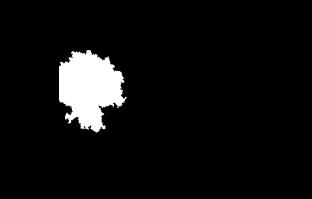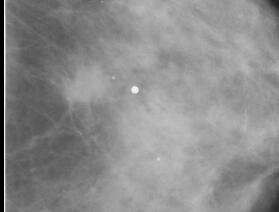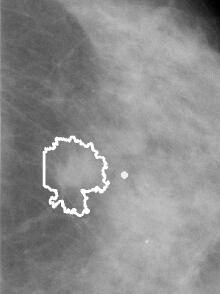展开全文•  L = bwlabel(BW,n) 返回一个和BW大小相同的L矩阵，包含了标记了BW中每个连通区域的类别标签，这些标签的值为1、2、num（连通区域的个数）。n的值为4或8，表示是按4连通寻找区域，还是8连通寻找，默认为8。 4连通...
https://blog.csdn.net/wanrenwangxuejing/article/details/25108191
用法：
L = bwlabel(BW,n)    返回一个和BW大小相同的L矩阵，包含了标记了BW中每个连通区域的类别标签，这些标签的值为1、2、num（连通区域的个数）。n的值为4或8，表示是按4连通寻找区域，还是8连通寻找，默认为8。    4连通或8连通是图像处理里的基本感念：而8连通，是说一个像素，如果和其他像素在上、下、左、右、左上角、左下角、右上角或右下角连接着，则认为他们是联通的；4连通是指，如果像素的位置在其他像素相邻的上、下、左或右，则认为他们是连接着的，连通的，在左上角、左下角、右上角或右下角连接，则不认为他们连通。请注意“或”字的含义，就是满足其中一个条件就认为是连通的。

[L,num] = bwlabel(BW,n)这里num返回的就是BW中连通区域的个数。

通俗的说，这个函数的作用是用来找这个二值图像中的连通区域的，对于不同的符合条件的连通区域（4连通，8连通）分别用不同的标号加以区别，结果保存在L这个矩阵里，而num里保存的是输入图像中连通区域的总数。
举例说明：     BW =     1     1     1     0     0     0     0     0     1     1     1     0     1     1     0     0     1     1     1     0     1     1     0     0     1     1     1     0     0     0     1     0     1     1     1     0     0     0     1     0     1     1     1     0     0     0     1     0     1     1     1     0     0     1     1     0     1     1     1     0     0     0     0     0     按4连通计算，方形的区域，和翻转的L形区域，有用是对角连接，不属于连通，所以分开标记，连通区域个数为3，就是有3个不同的连接区域。     L = bwlabel(BW,4)     结果如下：     L =     1     1     1     0     0     0     0     0     1     1     1     0     2     2     0     0     1     1     1     0     2     2     0     0     1     1     1     0     0     0     3     0     1     1     1     0     0     0     3     0     1     1     1     0     0     0     3     0     1     1     1     0     0     3     3     0     1     1     1     0     0     0     0     0     而8连通标记，它们是连通的：     [L, num] = bwlabel(BW,8)     L =     1     1     1     0     0     0     0     0     1     1     1     0     2     2     0     0     1     1     1     0     2     2     0     0     1     1     1     0     0     0     2     0     1     1     1     0     0     0     2     0     1     1     1     0     0     0     2     0     1     1     1     0     0     2     2     0     1     1     1     0     0     0     0     0    这里    num =2
展开全文• 背景：连通性分析，判断当前邻接矩阵W可以划分成几个互不联通的子图 如果图可以划分成2个互不联通的子图，那么其邻接矩阵W通过行列交换变换以后应该可以划分为[A,0;0,B]这种形式,其两个互不联通的子图的邻接矩阵...邻接矩阵
• 适合二值图像，通过自动扫描提取种子点，注释详细 参考文献：一种二值图像连通区域标记的新方法 陈柏生著。
• Matlab实现连通域标记算法求图像连通域连通域连通域标记算法 连通域 连通区域（Connected Component）一般是指图像中具有相同像素值且位置相邻的前景像素点组成的图像区域（Region，Blob）。连通区域分析（Connected...


Matlab实现连通域标记算法求图像连通域
连通域连通域标记算法

连通域
连通区域（Connected Component）一般是指图像中具有相同像素值且位置相邻的前景像素点组成的图像区域（Region，Blob）。连通区域分析（Connected Component Analysis,Connected Component Labeling）是指将图像中的各个连通区域找出并标记。 连通区域分析是一种在CVPR和图像分析处理的众多应用领域中较为常用和基本的方法。例如：OCR识别中字符分割提取（车牌识别、文本识别、字幕识别等）、视觉跟踪中的运动前景目标分割与提取（行人入侵检测、遗留物体检测、基于视觉的车辆检测与跟踪等）、医学图像处理（感兴趣目标区域提取）、等等。也就是说，在需要将前景目标提取出来以便后续进行处理的应用场景中都能够用到连通区域分析方法，通常连通区域分析处理的对象是一张二值化后的图像。
连通域标记算法
选用图像：A=imread('d:\Temp\test.png');
[m,n]=size(A);
B=zeros(m,n);%标记矩阵
label=1; %不同连通域标记值
q=zeros(9999,2);%模拟队列
tail=1;
neighbour=[-1,-1;-1,0;-1,1;0,-1;0,1;1,-1;1,0;1,1];  %与某像素点相加得到该像素点的邻域像素点

for j=1:n
for i=1:m
if A(i,j)~=0&&B(i,j)==0
B(i,j)=label;
q(tail,:)=[i,j]; %用元组模拟队列，当前坐标入列
tail=tail+1;

for k=1:8
pix1=pix+neighbour(k,:);
if pix1(1)>=1&&pix1(1)<=m&&pix1(2)>=1&&pix1(2)<=n
if A(pix1(1),pix1(2))~=0&&B(pix1(1),pix1(2))==0
B(pix1(1),pix1(2))=label;
q(tail,:)=[pix1(1) pix1(2)];
tail=tail+1;
end
end
end
end
label=label+1;
tail=1;
end
end
end
imshow(mat2gray(B));


代码效果：展开全文图像识别 算法
• 保留最大连通区域后，如何进行孔洞填充，孩子没学过多少matlab，简单解法就好有问必答
• 用法：  L = bwlabel(BW,n)  返回一个和BW大小相同的L矩阵，包含... 4连通或8连通是图像处理里的基本感念：而8连通，是说一个像素，如果和其他像素在上、下、左、右、左上角、左下角、右上角或右下角连接着，则认
• 解决微波仿真论坛中《Matlab与Feko软件混合》的几个小问题： . 找不到prefeko和runfeko程序； . 程序运行为单核模式，对于我们高性能服务器来说，造成了资源浪费； .模型只能整体移动，不能只针对...
• %显示最大连通区域， [~, max_id] = max(areas); max_rect = rects(max_id, :); % show the largest connected region % figure(2), % imshow(bw_img); % rectangle('position', max_rect, 'EdgeColor', 'r'); bw...图像分割
• 6.画出连通区域 7.连通域面积 8.找到每个区域对应的坐标值 9.只剔除连通域，不剔除矩形框 10.取行数 11.绘制矩形 如图： 通过更改se=strel(‘disk’,1)和bwareaopen(bw_img,50,8)的值来腐蚀膨胀去除较小的连通域，8...连通域检测
• 关于stk与matlab的版本对应关系以及一般正确的连接方式请看谈谈AGI STK跟matlab的互联问题。通过看这篇文章，我重新下载了matlab和stk的版本，使用了STK11和matlab2015b，在STK端查看edit-preference-matlab都没有...
• 通信网基础仿真实验 图的连通性判断 matlab语言
• 根据邻接矩阵求最大连通分支的MATLAB函数，输入为邻接矩阵，输出为最大连通分支点集。
• grayimg = rgb2gray(flag_yellow); % 灰度图像 % grayimg 的色彩空间为 Gray BWimg = grayimg; [width,height]=size(grayimg); % 二值化 BWimg = im2bw(grayimg, 0.8);...%分割出连通域内的图像opencv 计算机视觉 python 算法
• 基于联通区域的matlab图像分割，对提取树叶上的害虫等的轮廓或纹理特征有独到的效果图像分割
• 8连通，是说一个像素，如果和其他像素在上、下、左、右、左上角、左下角、右上角或右下角连接着，则认为他们是联通的； 4连通，是指如果像素的位置在其他像素相邻的上、下、左或右，则认为他们是连接着的，...
• 最近motion graph相关实验，发现实现运动过渡需要构建运动图，而为了避免运动过渡陷入死胡同，需要对图结构进行裁剪，方法就是计算图模型的极大强联通分量，但是自己懒得去实现，所以就去搜了一下matlab中有无相关...
• 这个代码功能是随机的生成20个结点，并随机选择其中的某些点进行连接， 表示相互间有通信，最终生成连通图。并将邻接矩阵和拉普拉斯阵输出成.txt文档。
• 各位大佬们，我在阈值分割后得到了各个连通区域的参数，现在我想只对圆形的连通区域用最小二乘法来拟合标准圆，怎么排除其他连通区域的干扰啊，，求指教啊
• matlab函数_连通区域 1、 matlab函数bwareaopen──删除小面积对象 格式：BW2 = bwareaopen(BW,P,conn) 作用：删除二值图像BW中面积小于P的对象，默认情况下使用8邻域。 算法： (1)Determine the connected ......

# matlab连通matlab 订阅